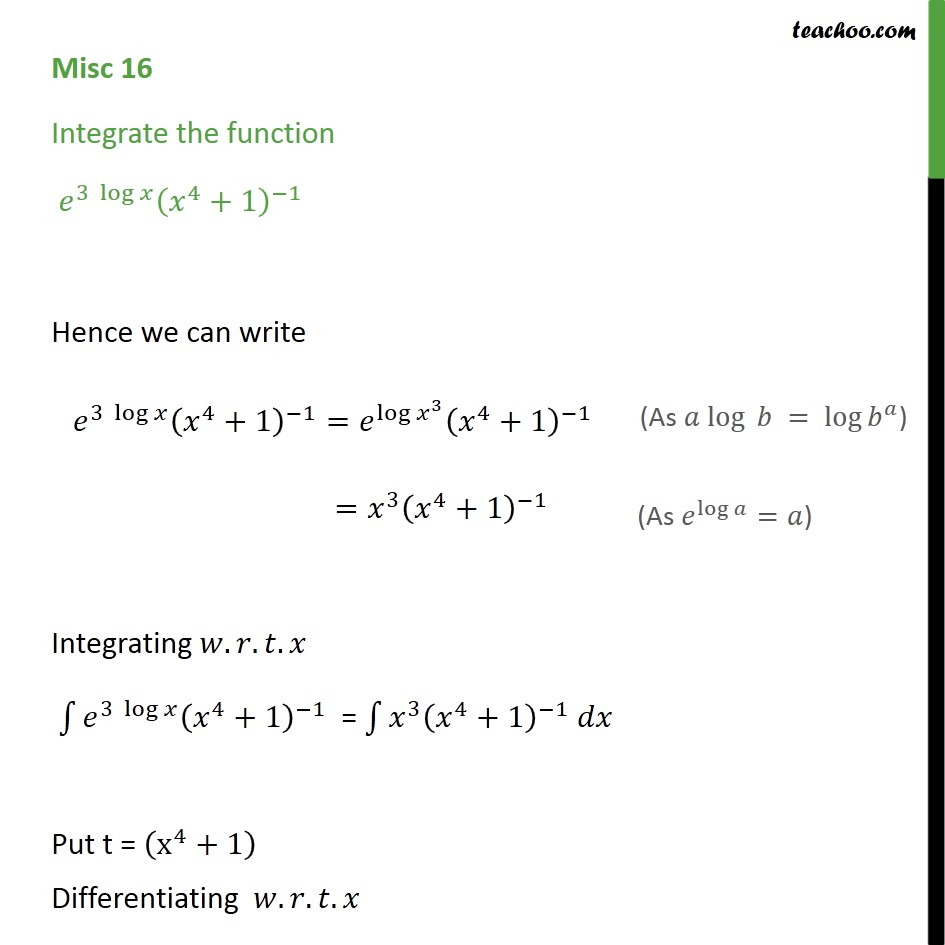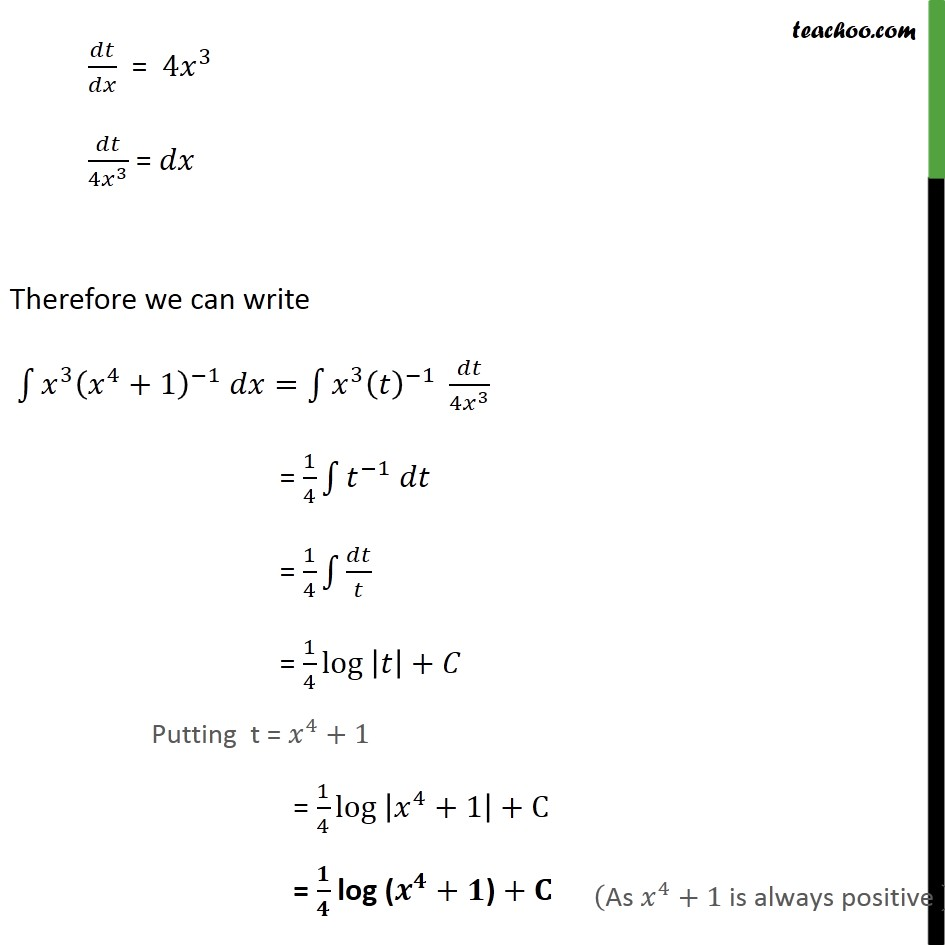Miscellaneous

Chapter 7 Class 12 Integrals
Serial order wiseLearn in your speed, with individual attention - Teachoo Maths 1-on-1 Class

### Transcript

Misc 16 Integrate the function 3 log 4 +1 1 Hence we can write 3 log 4 +1 1 = log 3 4 +1 1 = 3 4 +1 1 Integrating . . . 3 log 4 +1 1 = 3 4 +1 1 Put t = x 4 +1 Differentiating . . . = 4 3 4 3 = Therefore we can write 3 4 +1 1 = 3 1 4 3 = 1 4 1 = 1 4 = 1 4 log + Putting t = 4 +1 = 1 4 log 4 +1 +C = log ( + ) +